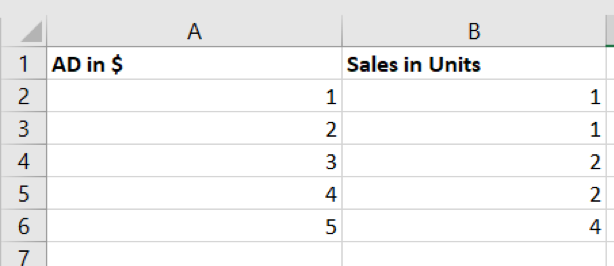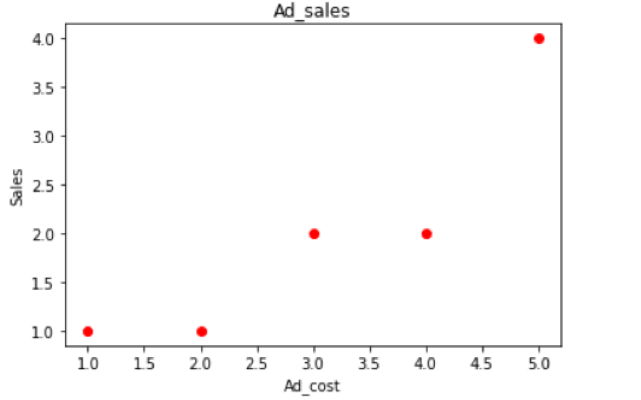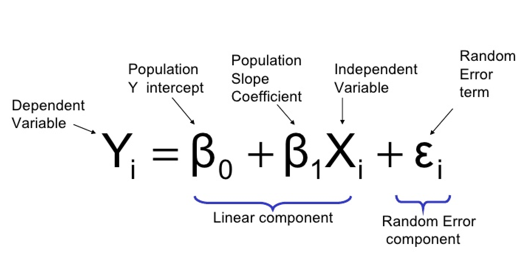Search

# All You Need to Know About Linear Regression (Part I)

This article is written with the intention of simplifying our understanding of the theoretical and practical implications of regression techniques in the field of marketing. We will subsequently post Python and R codes to illustrate relevant examples.

Let us go over each and every section thoroughly and understand techniques for regression. We have Mr Question with us, who loves to ask questions. Handing over to Mr Question.

Why to use Regression?

1. Data description

2. Parameter estimation

3. Prediction and estimation

4. Control

“Regression involves prediction of the response variable. For example, we may wish to predict delivery time for a specified number of cases of Coca-Cola to be delivered. These predictions may be helpful in planning delivery activities such as scheduling or in evaluating the productivity of delivery operations.”

### What is Linear Regression?

Regression - In layman terms, regression estimates a relationship between dependent and independent variables.

Linear Regression – When there is a linear relationship between dependent and independent variables, it’s called linear regression.

Mr Question: Wait, that’s it?—I thought there was more to it! So, what are dependent and independent variables?

Sales in Units: I am dependent. As AD in \$ changes, I change. I trust the independent variable(s) to provide me guidance.

AD in \$: I am independent because I do not depend on anyone. If you find me correlating with someone then make sure you check your model for multicollinearity!Table 1 shows us two columns AD in \$ and Sales in Units.

Mr Question: Multicollinearity? What is that?

Multicollinearity- I appear when I find a relationship between X or independent variables. In this example, you have one X or independent variable so do not panic. However, always remember to keep a look out for me when there are multiple independent variables!

Mr Question: But how can I see that?

Variance Inflation Factor- If you find me having a value of more than 5 or 10, then you can be sure your independent variables have correlations. If I am equal to 1, then there is no multicollinearity.

Mr Question: How to deal with it?

Principal component analysis (PCA): I am here to deal with it. We will understand you later, Mr Dimensionality Reduction. We will attend your magic show!!

Let us cover some functions of PCA

1. Dimension reduction

2. A linear transformation

3. Uses statistics and calculus

4. Visualization algorithm, you can visualize high dimensions in low dimensions

PCA is for linear, similarly we can use t-SNE for non-linear.

t-SNE: t- distributed Stochastic Neighbour Embeddings, from the name we can say it must be using t-test. Another way to remove highly correlated predictors from the model.

Mr Question: In simple linear regression, there will be only one input and one output. The output should be a continuous variable. Now, what is continuous? Continuous variables are measurements on a continuous scale, or a quantitative variable measured in some way. Examples include weight, time and length.

Let us start our topic in this way!

You are a marketing analyst in a toy store and data on advertisement cost and sales in unit as seen above.

AD in \$: I am X, you can also call me as independent variable or regressor variable.

Sales in Units: I am Y, I can be termed as dependent variable or response variable.

Scatter plot: I will help you guys investigate the relationships between variables.Scatterplot designed from Table 1

Equation of Regression: Look at me guys!Given a vector of inputs XT = (X1,X2, . . . ,Xp), we predict the output Y via the model. β 0 is bias in machine learning. The linear model in vector form as an inner product isEquation of Simple Linear Regression:Where the intercept (β0) and the slope (β 1) are unknown constants and (ε) is a random

error component.

### Assumptions

Random Error Term or Epsilon (ε): I am random variable with zero mean and variance (σ 2 ). I am irreducible or commonly known as a measurement error.

Y (response variable): I am also random variable.

X (regressor): I am constant, I do not depend on others. I am independent.

So, Probability distribution for y at each possible value for x.

The mean of distribution is E(y| x) = β0 +β1x

And variance is Var(y| x) = Var (β0 +β1x +ε ) = σ 2

Variance: Why am I here?

Random Error (ε): Because I am you have me in the equation and as you know expected of (random error) is 0, so in mean of distribution E(y| x)= β0 +β1x

Mr Question: So, what is this equation for? And why do we require this equation?

New points: As we have data X and Y, we need to predict Y or dependent variable for new points of X or independent variable. You can consider infinite number of functions but need to choose the best possible one. Linear regression is one such function.

Mr Question: Now what is β0, β1 ? (who are you guys and what are you doing here)?

β 0, β 1: We're both parameters or coefficients.

β 0: I am intercept.

β 1: And I’m slope!

Mr Question: So, it means that the parameters β 0 and β 1 are unknown and must be estimated using sample data.

### Estimation of Parameters

Least squares: Use me to estimate β 0 (intercept) and β 1 (slope). I will help you, with the analytical way!

Mr Question: THE ANALYTICAL WAY?

Least squares: Yes, for numerical approximation use Residual Sum of Squares.

Residual Sum of Squares:Mr Question: So, who are you?

Residual Sum of Squares: I’m the difference between actual output and predicted output. In the above equation is the actual output from the data and (β0 + β1*xi ) is giving the predicted output.

Mr Question: Where do you get actual output?

Actual Output: I am in the data itself. In above table, I am known as Y or dependent variable.

Mr Question: But where are we getting predicted output? In linear regression, predicted output is measured from the equation (yi = β0 + β1 *xi ). Our intention is to find β0 and β1.

Partial derivative: I will help least squares! Let us use partial derivative with respect to β 0 and β 1 in S or above equation.As for :Dividing n to each part we will get the equation,This is the equation for bias or intercept. So ȳ and are the mean of Y and X respectively. How have we got and ȳ in the equation?

From the below equations:So finally, we got our β0

Put β0 equation inYAY! So, we found out β0 and β1.

The difference between truth or actual value and fitted value is called residual (ε). Finally, we’re done with our equations!

Mr Question: Usually a question arises why we use L2 norm or sum of squares instead of L1 norm or absolute values. We can use absolute right then why to use L2 norm?

Norms: In simple words, we want to punish the points that are further from the regression line much more than the points which lie close to the line. Usually square distances make easier to derive a regression line. Also, L2 norm helps to increase the error distance and helps to reflect bad predictions.

Mr Question: Hmm regression line? How to get it, and what is that? Who are you?

Regression line: I am the best fit linear line in the linear regression scatter plot. I will give you the line when you put the values of and in your Linear Regression Equation. is the intercept, where the line starts.

β0: Regression line starts from me.

Regression line: If you are not there or zero, I will start from origin and the regression will be named as univariate regression.

Mr Question: OHHH!! Nice.

### Test Significance

Mr Question: In code we can see (***) in the R codes and can interpret independent variable is highly significant with dependent variable. But how can we do so manually?If we fail to reject H 0 : β 1 = 0, then there is no relationship between independent and dependent variable or true relation between independent and dependent variable is not linear.### Testing Model

1. T-testAnd | t 0 | > t α /2, n − 2

t α /2, n − 2 value can be drawn from t table, where n-2 is degree of freedom.MSRes is Mean Square Residual2. ANOVA

ANOVA: We use a f-test here, so make sure you use my table.

Mr Question: Sure, we love your simplified table. Here we go.In above table, Total = Regression + Residual.

Degrees of Freedom (DF) of Regression is 1 because, the formulae is K-1, here k is 2 as we have 1 dependent and 1 independent variable.

Reject , whenSimilarly, use F table to get F α ,1, n−2. We want to see how confident we are in our model. Always remember to check low variance and high bias in your model!

Mr Question: Low variance and high bias?

Linear Regression- You need to check bias and variance. Everyone knows me for low variance and high bias. I am low in flexibility but high in interpretability.

Mr Question: So, what’s low variance and high bias?

Low Variance: Under repeated sampling, the line will stay roughly in the same place.

High Bias: Average of the models cannot get true relationship.

Confidence Interval: I am here!!

If we get 95% CIs on the slope for each sample, then 95% of the intervals will contain the true value of β1.

i.e. 100 * (1 − α ) percent CI

We can also interpret this as we reject the null hypothesis it means there is a relation between dependent and independent variables. We also can infer that 95% C.I. does not include zero.

P-Value: Do not forget me! If I am less than 0.05, reject the Null Hypothesis. I can assure you that both dependent and independent variables have a relation.

### Evaluating Model

Mr Question: Once we done with the above procedures, one question arises how well the model describes the data? Again, we can use 2 methods.

1. Root Mean Square Error (RMSE)

RMSE: Did anyone mention my name?

Mr Question: Yes—you are the one who can evaluate our model and say how much error between the actual output and predicted outputMr Question: Thanks!

2. R-Square: I am the coefficient of determination; I can explain total variability in your response model. Yes R-squared is a statistical measure of how close the data are to the fitted regression line and lies between 0 to 1. There is no error made when R2 (Rsquare) is 1. It means the higher the R-squared, the better the model fits your data. However, remember that I’m not the only metric you should look at when evaluating your model!

Mr Question: Wait, so a R-square with low value is not bad?

R-square: Not necessarily, no. Predicting human psychology is not easy so a low R-square value does not indicate the model is bad.

Written By: Siddhish Satapathy

Edited By: Varun Manoj